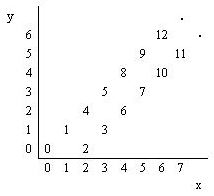# 1106. Number Steps

Starting from point (0,0) on a plane, we have written all non-negative integers 0,1,2, … as shown in the figure. For example, 1, 2, and 3 has been written at points (1,1), (2,0), and (3, 1) respectively and this pattern has continued.You are to write a program that reads the coordinates of a point (x, y), and writes the number (if any) that has been written at that point. (x, y) coordinates in the input are in the range 0…5000.

### 输入格式

The first line of the input is N, the number of test cases for this problem. In each of the N following lines, there is x, and y representing the coordinates (x, y) of a point.

### 输出格式

For each point in the input, write the number written at that point or write No Number if there is none.

### 样例

Input
3
4 2
6 6
3 4

Output
6
12
No Number


86 人解决，100 人已尝试。

99 份提交通过，共有 148 份提交。

2.4 EMB 奖励。